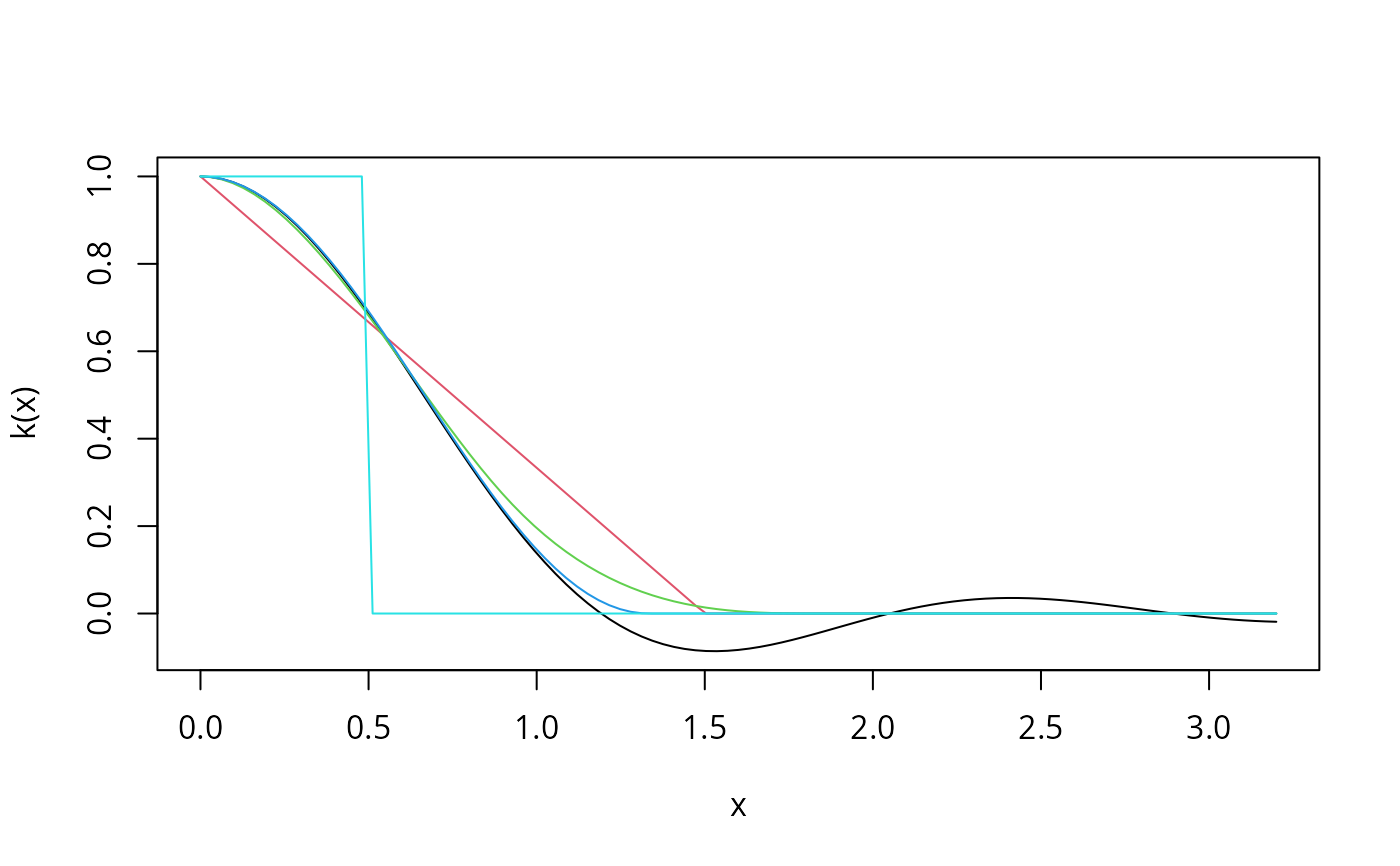Kernel weights for kernel-based heteroscedasticity and autocorrelation consistent (HAC) covariance matrix estimators as introduced by Andrews (1991).

kweights(x, kernel = c("Truncated", "Bartlett", "Parzen",
"Tukey-Hanning", "Quadratic Spectral"), normalize = FALSE)

## Arguments

x

numeric.

kernel

a character specifying the kernel used. All kernels used are described in Andrews (1991).

normalize

logical. If set to TRUE the kernels are normalized as described in Andrews (1991).

## Value

Value of the kernel function at x.

kernHAC, weightsAndrews

## Examples

curve(kweights(x, kernel = "Quadratic", normalize = TRUE),
from = 0, to = 3.2, xlab = "x", ylab = "k(x)")
curve(kweights(x, kernel = "Bartlett", normalize = TRUE),
from = 0, to = 3.2, col = 2, add = TRUE)
curve(kweights(x, kernel = "Parzen", normalize = TRUE),
from = 0, to = 3.2, col = 3, add = TRUE)
curve(kweights(x, kernel = "Tukey", normalize = TRUE),
from = 0, to = 3.2, col = 4, add = TRUE)
curve(kweights(x, kernel = "Truncated", normalize = TRUE),
from = 0, to = 3.2, col = 5, add = TRUE)